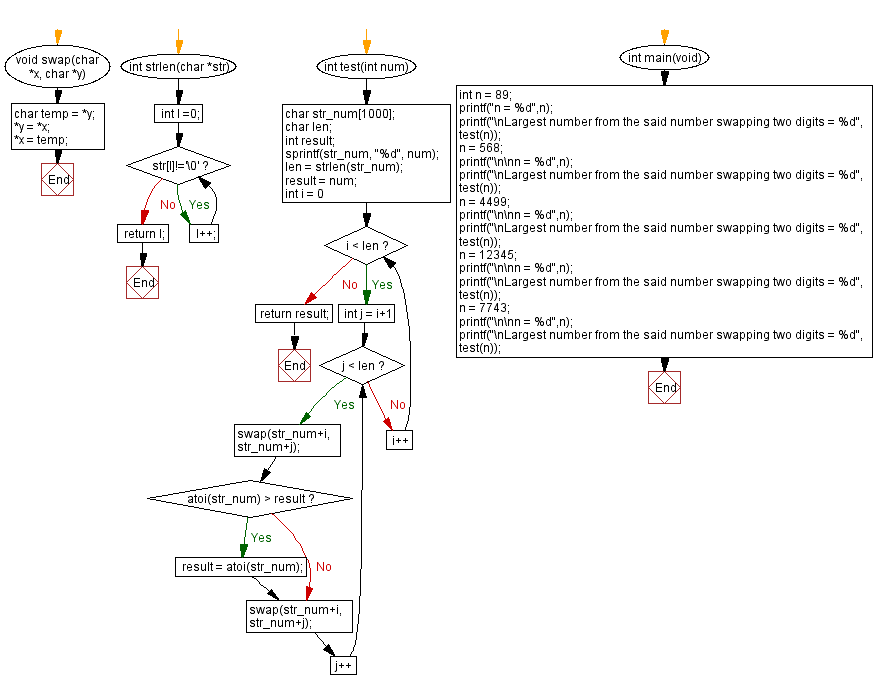﻿ C - Largest number, swapping two digits in a number

# C Programming: Largest number, swapping two digits in a number

## C Programming Mathematics: Exercise-31 with Solution

Write a C programming to calculate the largest number that can be generated by swapping just two digits at most once

Test Data:
(89) -> 98 [Swapping 8 and 9]
(568) -> 865 [Swapping 8 and 5]
(4499) -> 9494 [Swapping 9 and 4]
(12345) -> 52341 [Swapping 1 and 5]
(7743) -> 52341 [No Swap]

Sample Solution:

C Code:

``````#include <stdio.h>
#include <stdlib.h>
#include <string.h>
void swap(char *x, char *y) {
char temp = *y;
*y = *x;
*x = temp;
}

int strlen(char *str)
{
int l =0;
while(str[l]!='\0')
{
l++;
}
return l;
}

int test(int num){
char str_num;
char len;
int result;
sprintf(str_num, "%d", num);
len = strlen(str_num);
result = num;
for (int i = 0; i < len; i++) {
for (int j = i+1; j < len; j++) {
swap(str_num+i, str_num+j);
if (atoi(str_num) > result) {
result = atoi(str_num);
}
swap(str_num+i, str_num+j);
}
}
return result;
}
int main(void) {
int n = 89;
printf("n = %d",n);
printf("\nLargest number from the said number swapping two digits = %d", test(n));
n = 568;
printf("\n\nn = %d",n);
printf("\nLargest number from the said number swapping two digits = %d", test(n));
n = 4499;
printf("\n\nn = %d",n);
printf("\nLargest number from the said number swapping two digits = %d", test(n));
n = 12345;
printf("\n\nn = %d",n);
printf("\nLargest number from the said number swapping two digits = %d", test(n));
n = 7743;
printf("\n\nn = %d",n);
printf("\nLargest number from the said number swapping two digits = %d", test(n));
}
```
```

Sample Output:

```n = 89
Largest number from the said number swapping two digits = 98

n = 568
Largest number from the said number swapping two digits = 865

n = 4499
Largest number from the said number swapping two digits = 9494

n = 12345
Largest number from the said number swapping two digits = 52341

n = 7743
Largest number from the said number swapping two digits = 7743
```

Flowchart:C Programming Code Editor:

Improve this sample solution and post your code through Disqus.

What is the difficulty level of this exercise?

Test your Programming skills with w3resource's quiz.

﻿